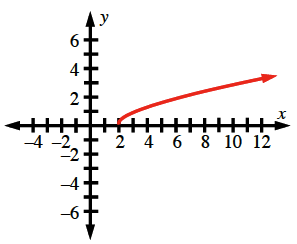### Home > CC3 > Chapter Ch9 > Lesson 9.2.4 > Problem9-119

9-119.

Make a table and graph the rule $y = \sqrt { x - 2 }$ that includes $x$‑values from $−1$ to $10$. Graph the rule on graph paper.

Make the table first. Then plot points using the table. You will have to use decimal approximations for many of the $y$-values in order to plot all the points. This rule does not form a line.

There will be no values for $x < 2$.